# Inter maths solutions for Complex numbers

Intermediate mathematics IIA Complex numbers exercises 1(a), 1(b), 1(c) and 1(d) textbook solutions are given.

These solutions are very easy to understand. Please study the textbook lessons well.

Then observe the example problems and solutions. Try them.

Observe the solutions given below. Try them in your own method.

You can also see the solutions

SSC Maths text book Solutions class 10

Inter maths 1A textbook solutions

Inter Maths 1B solutions

Inter Maths 1IA text book solutions

Inter Maths IIB text book solutions

Ncert maths class 6 chapter 14 solutions

Nios maths 311 book 1 Sets 1.1 solutions

Solutions for textbook exercises

# Inter maths solutions for textbook exercises Complex numbers

Exercise 1(a)## Complex numbers exercises solutions inter

Exercise 1(b)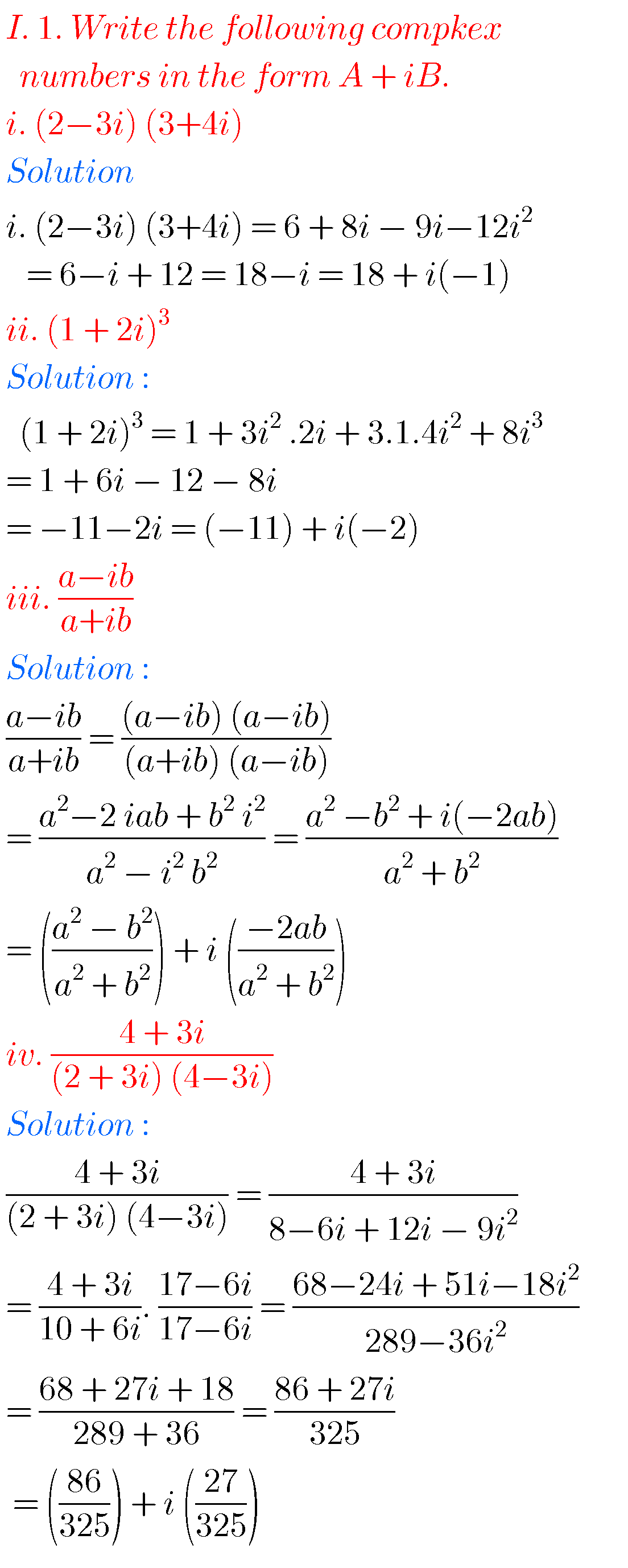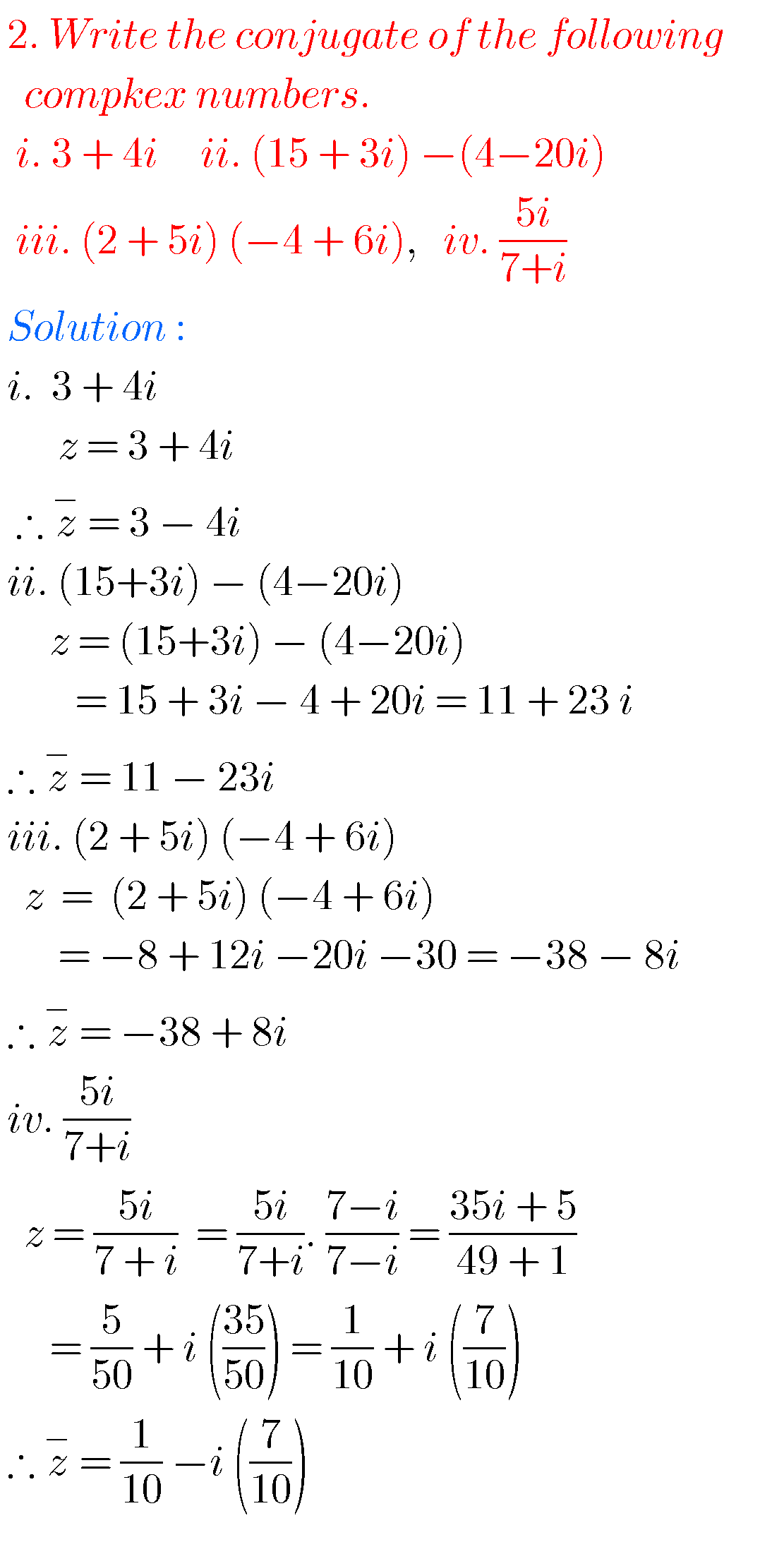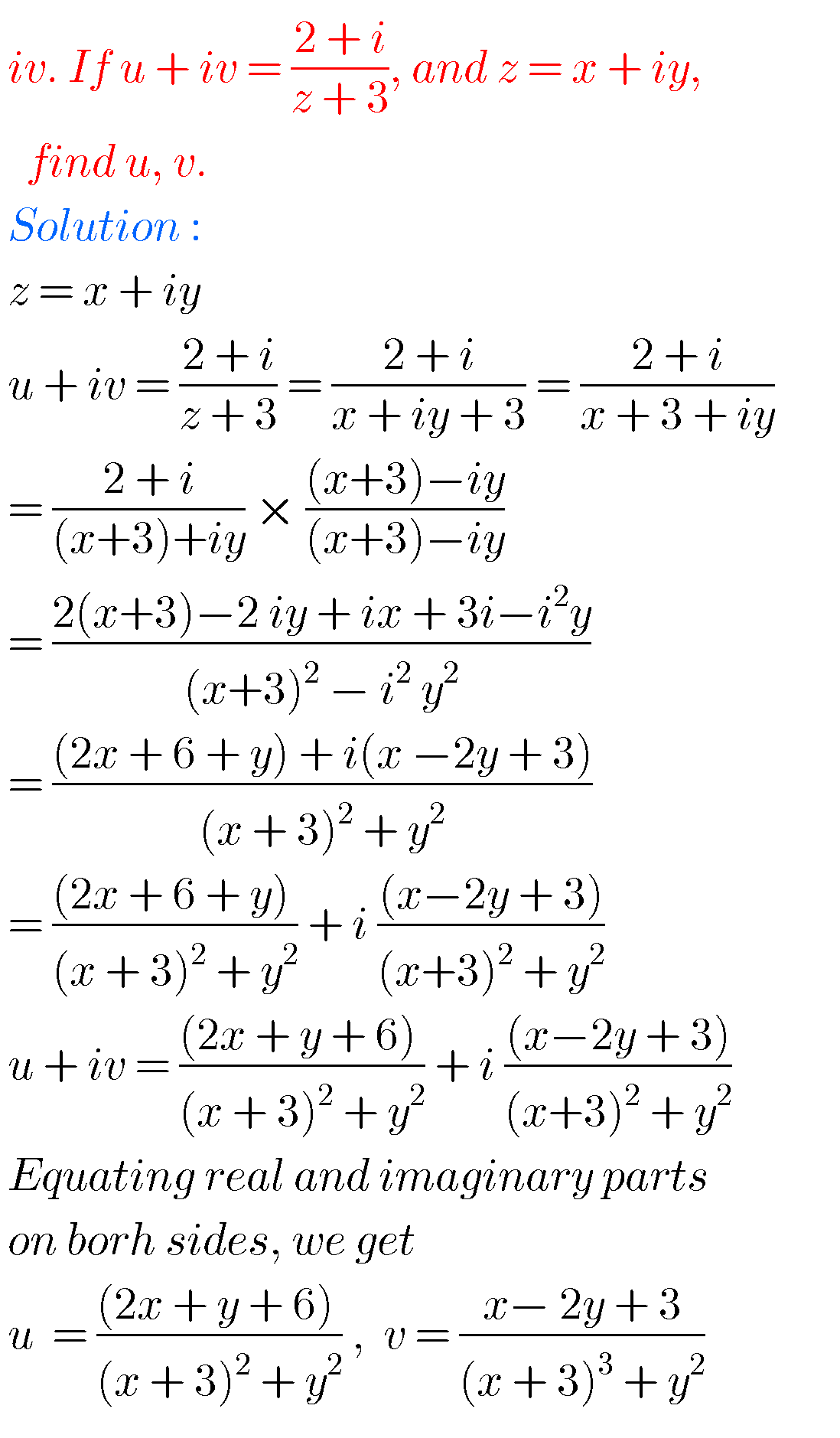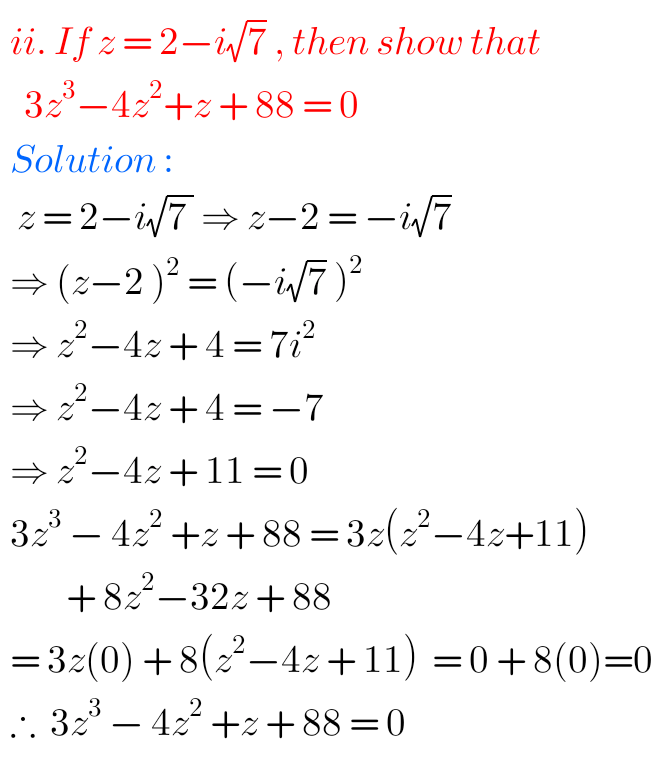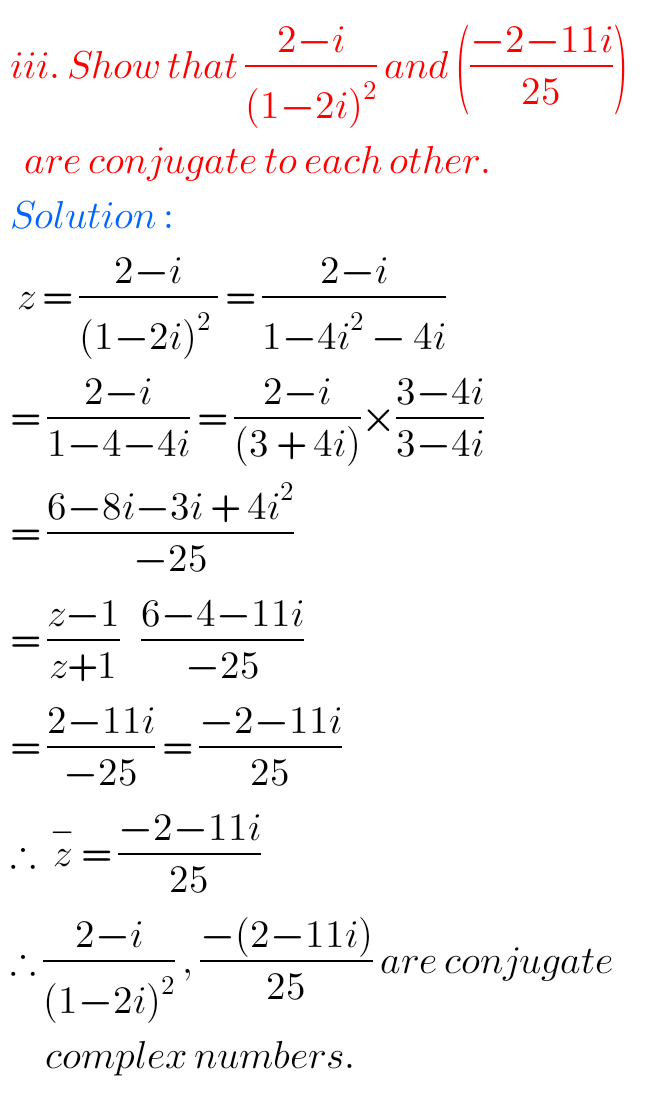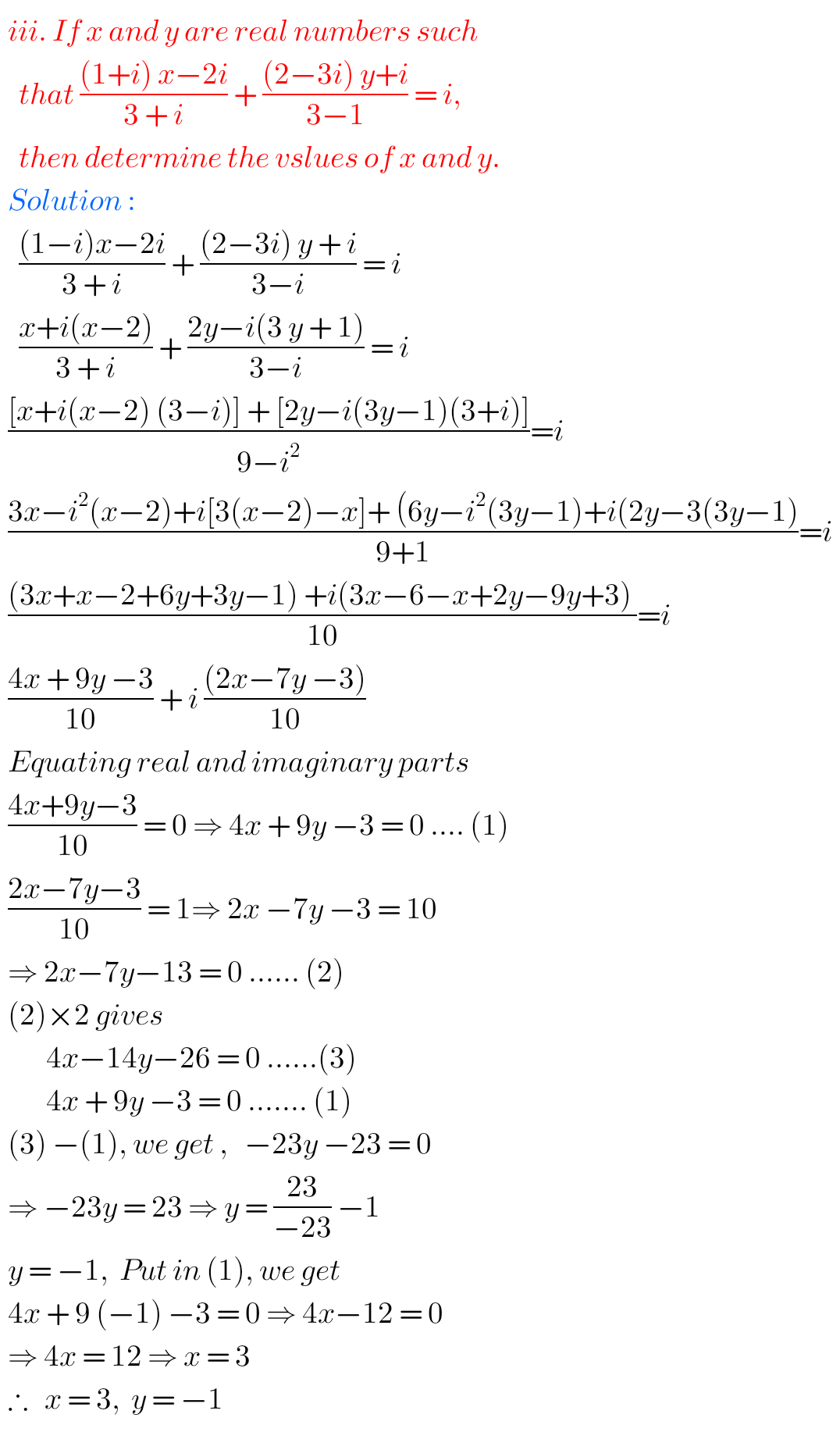## Solutions for mathematics Complex numbers inter textbook

Exercise 1(c)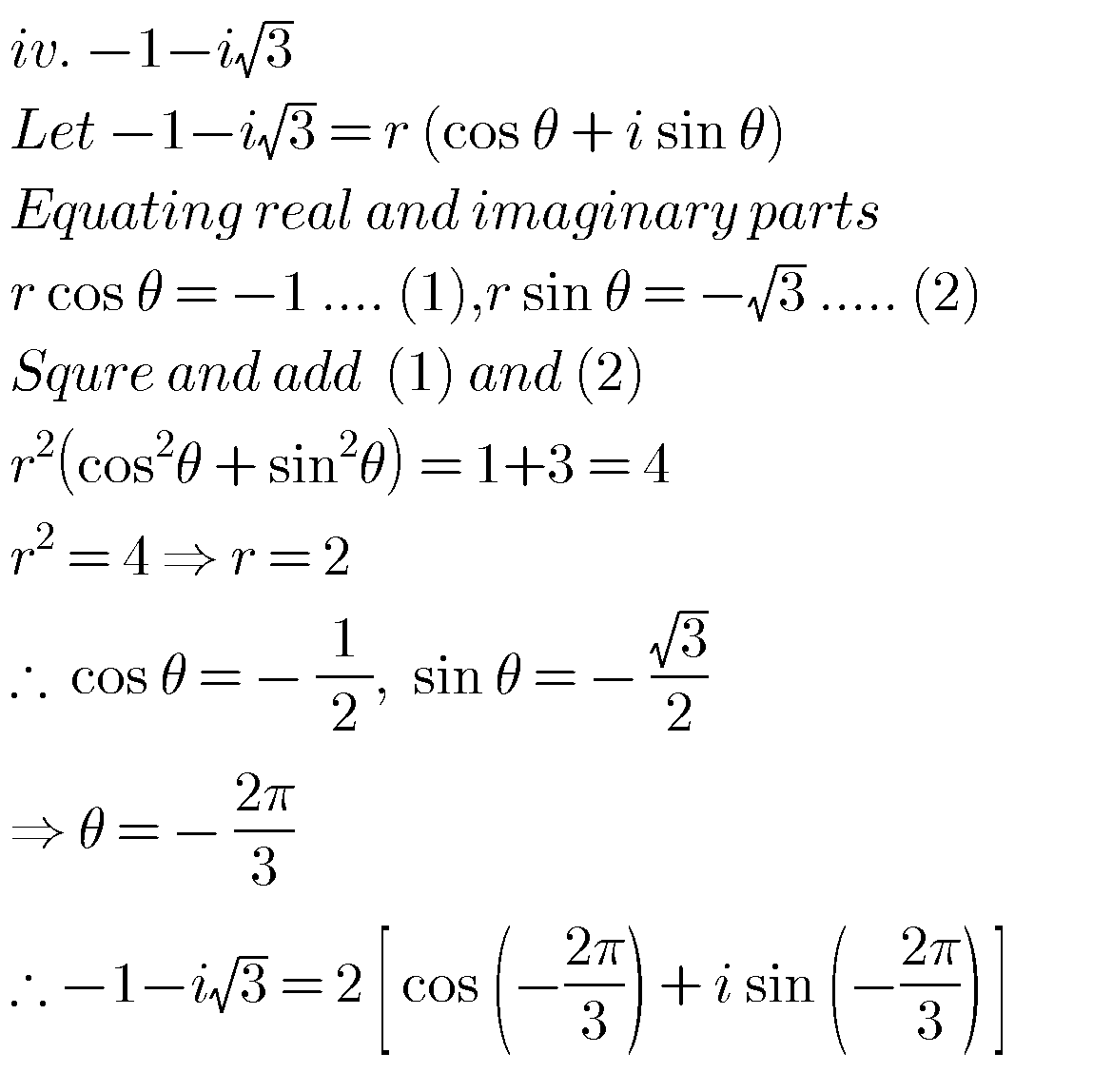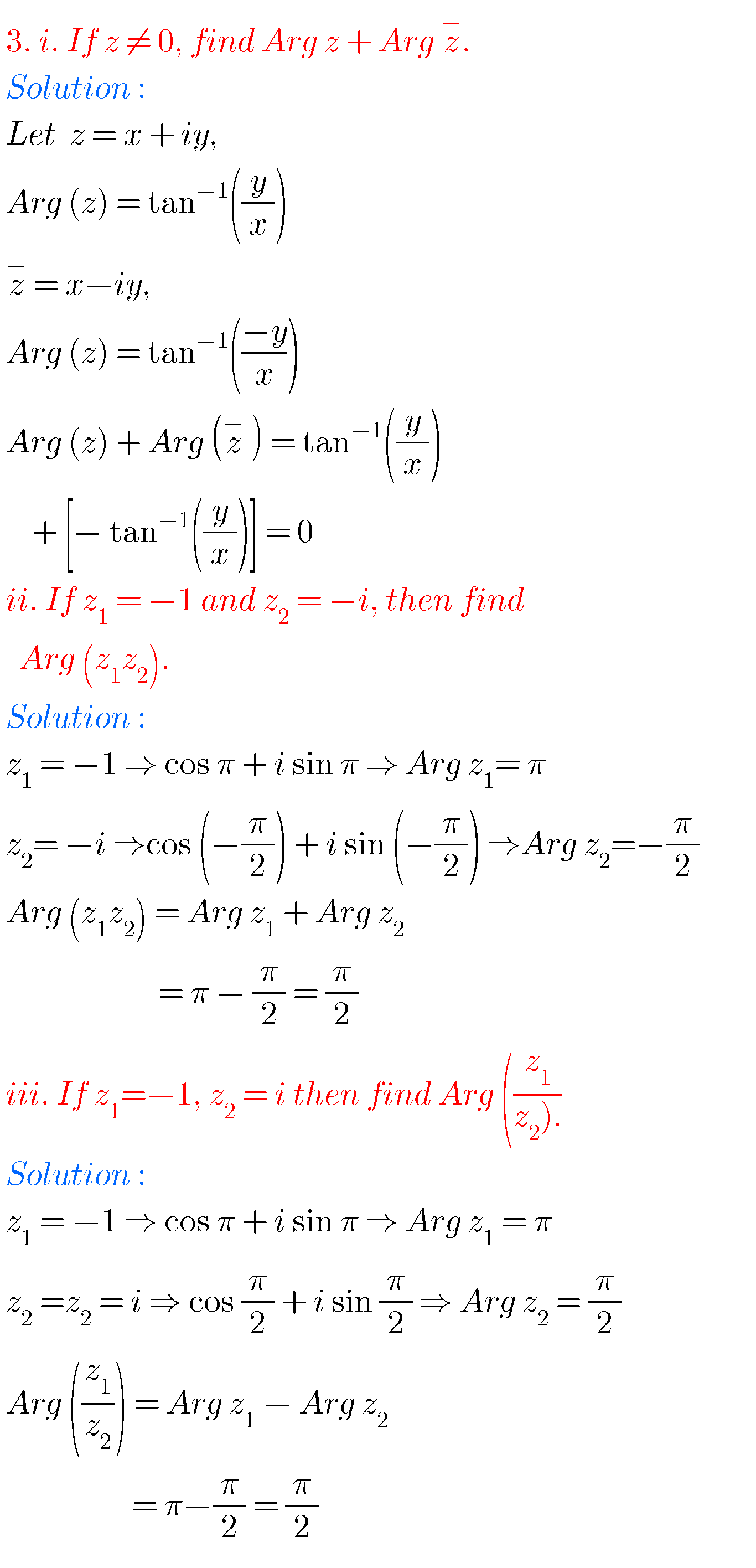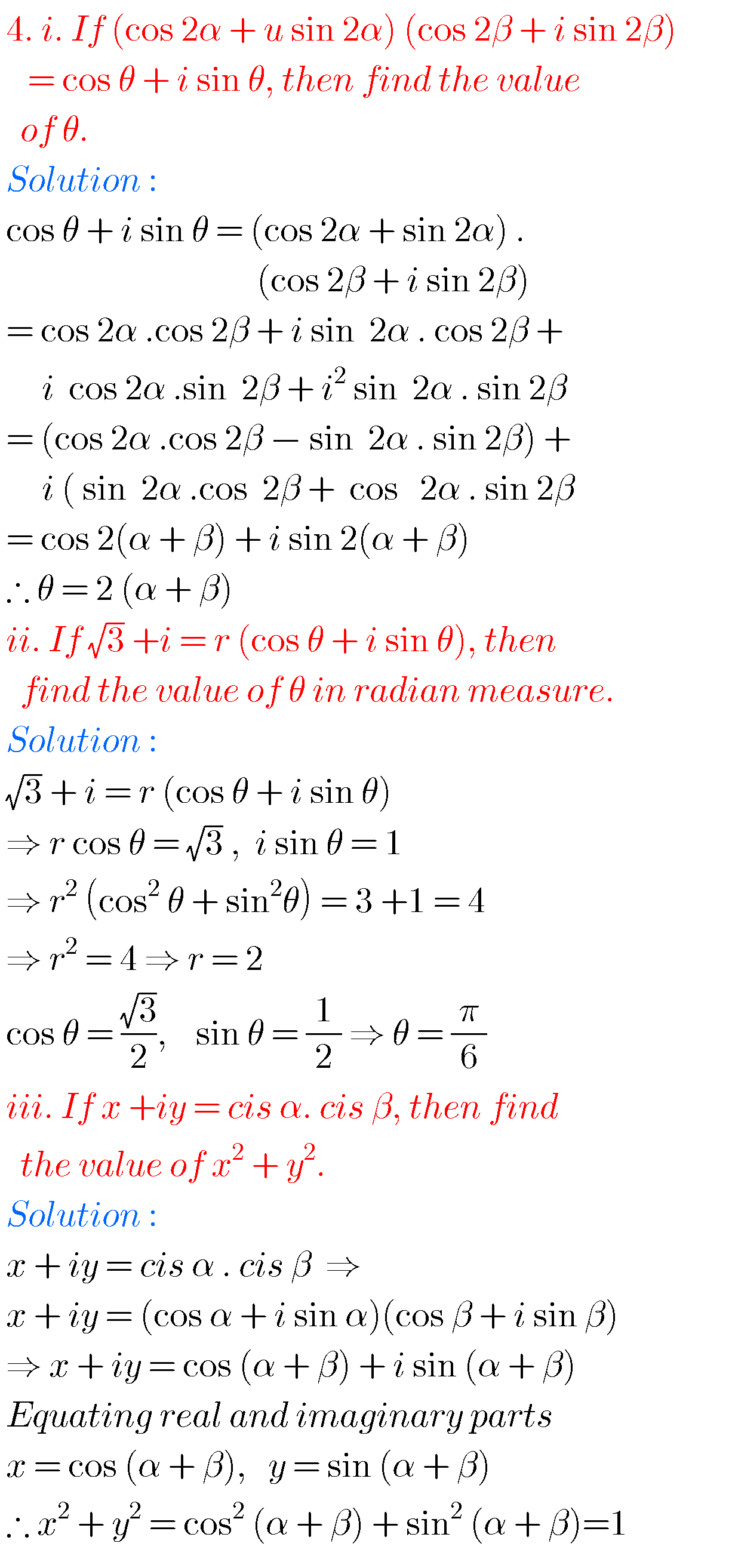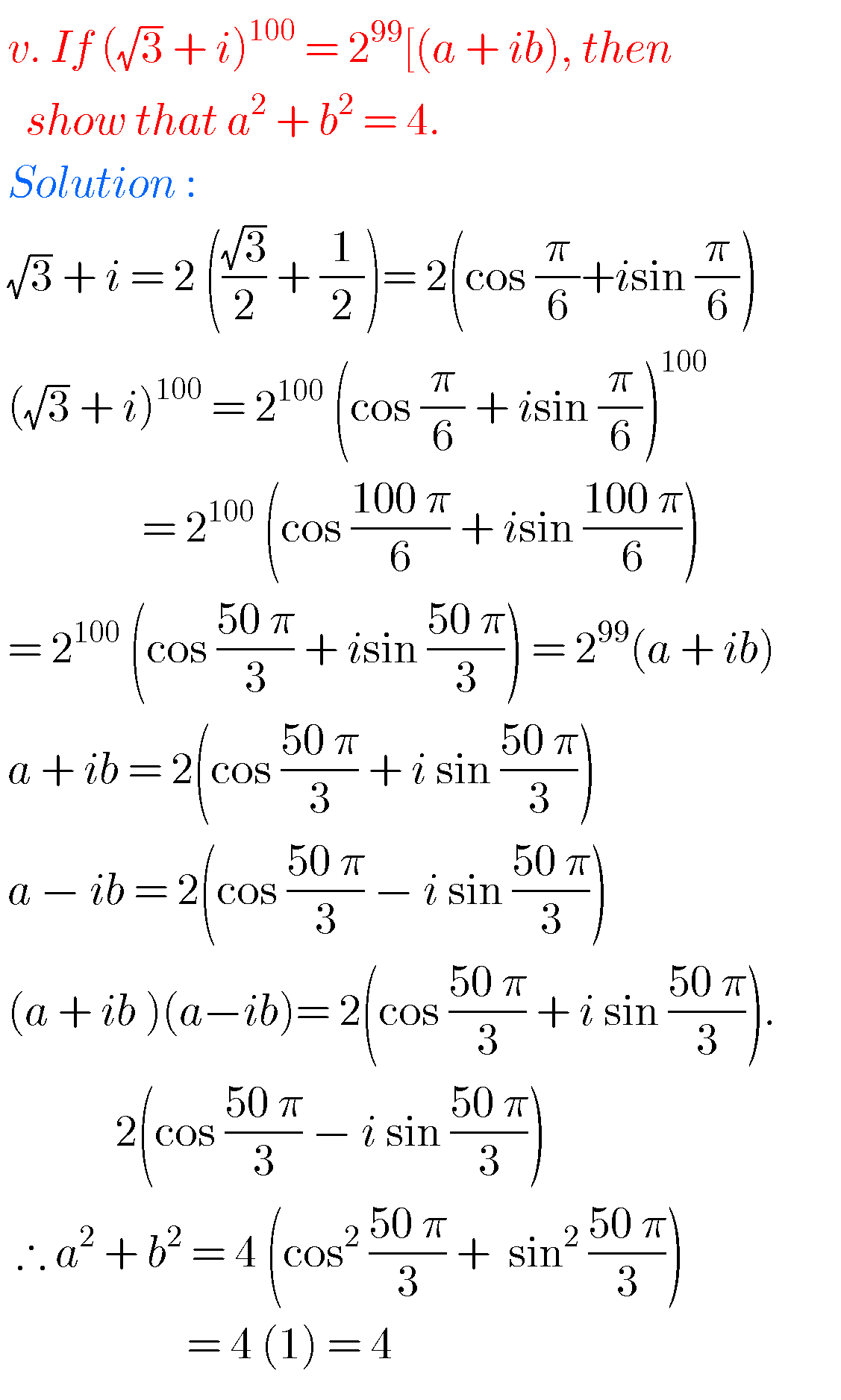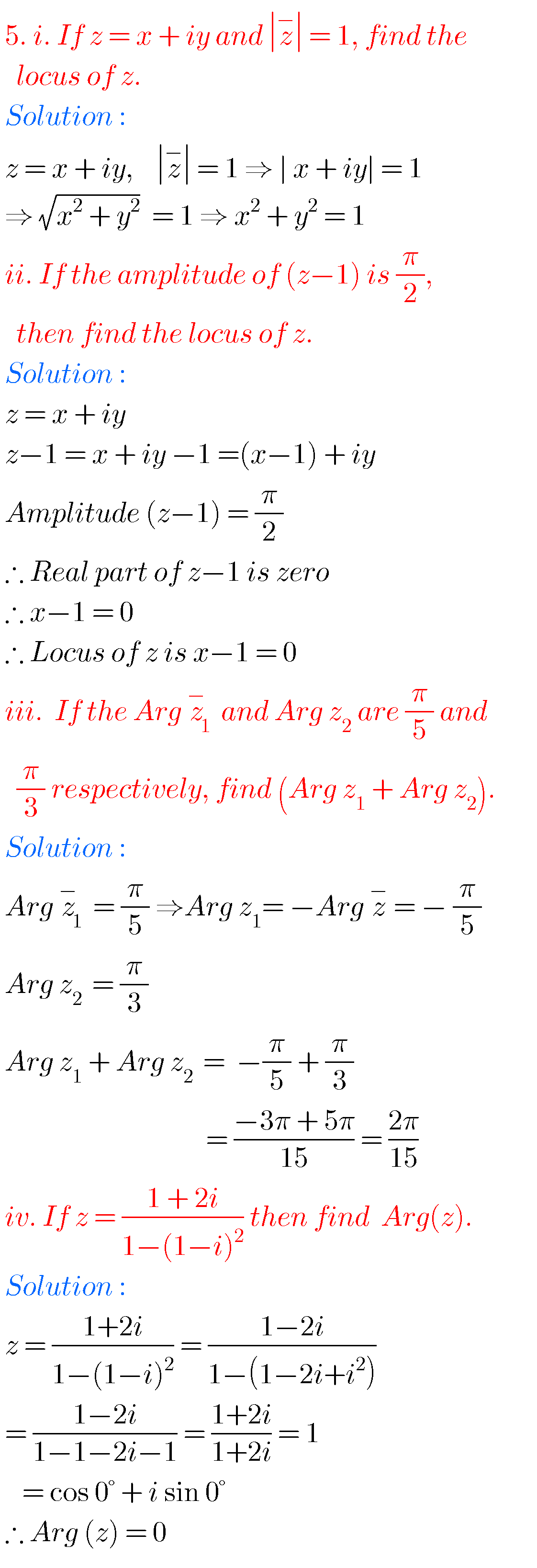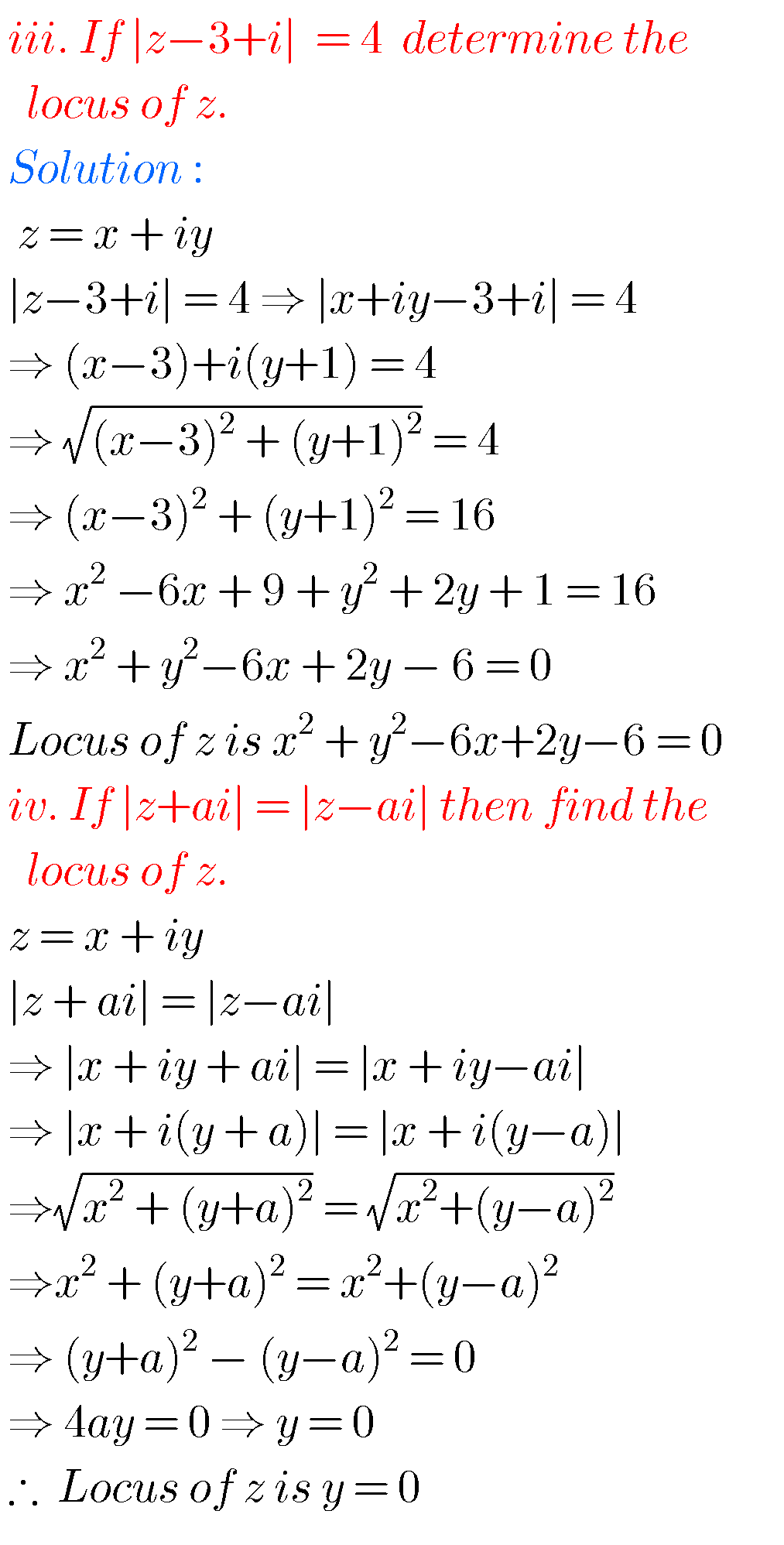### Textbook exercises solutions for Complex numbers inter 2a

Exercise 1(d)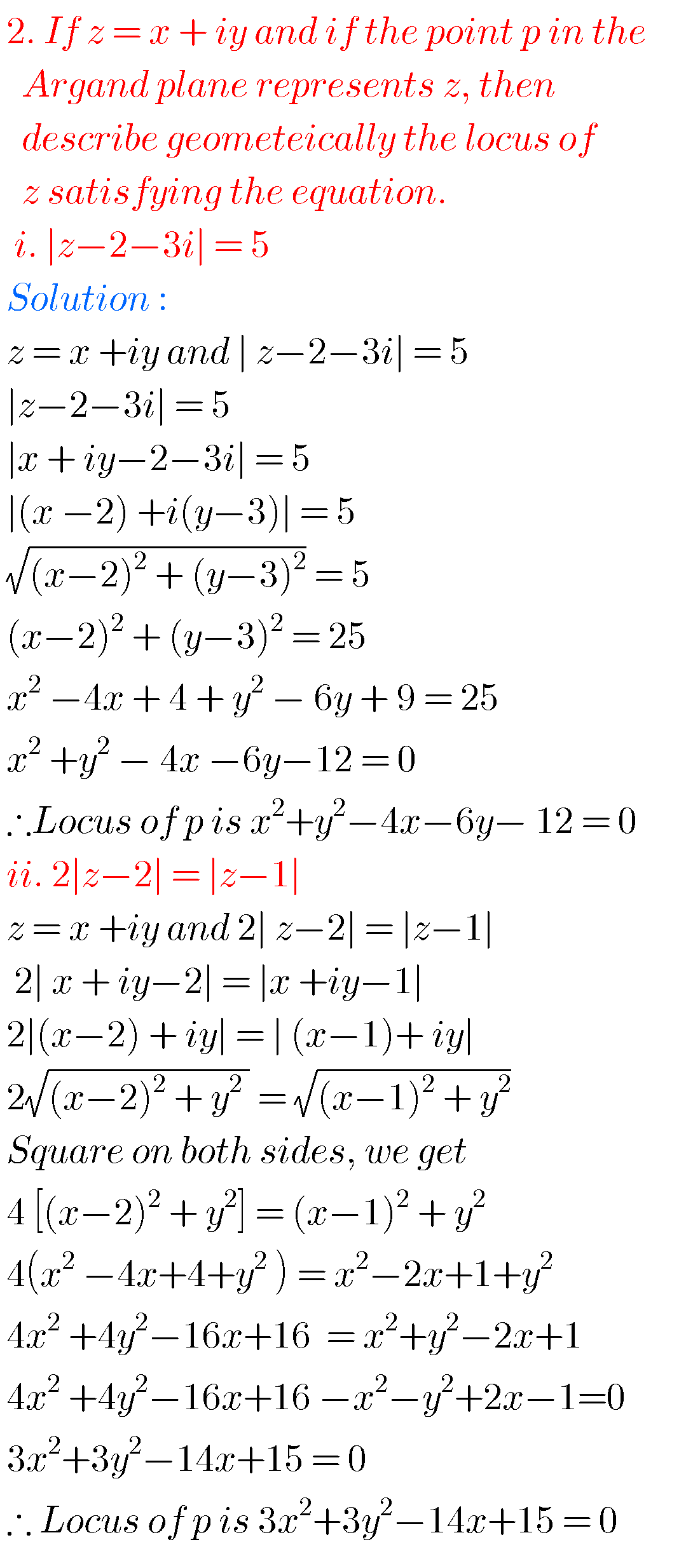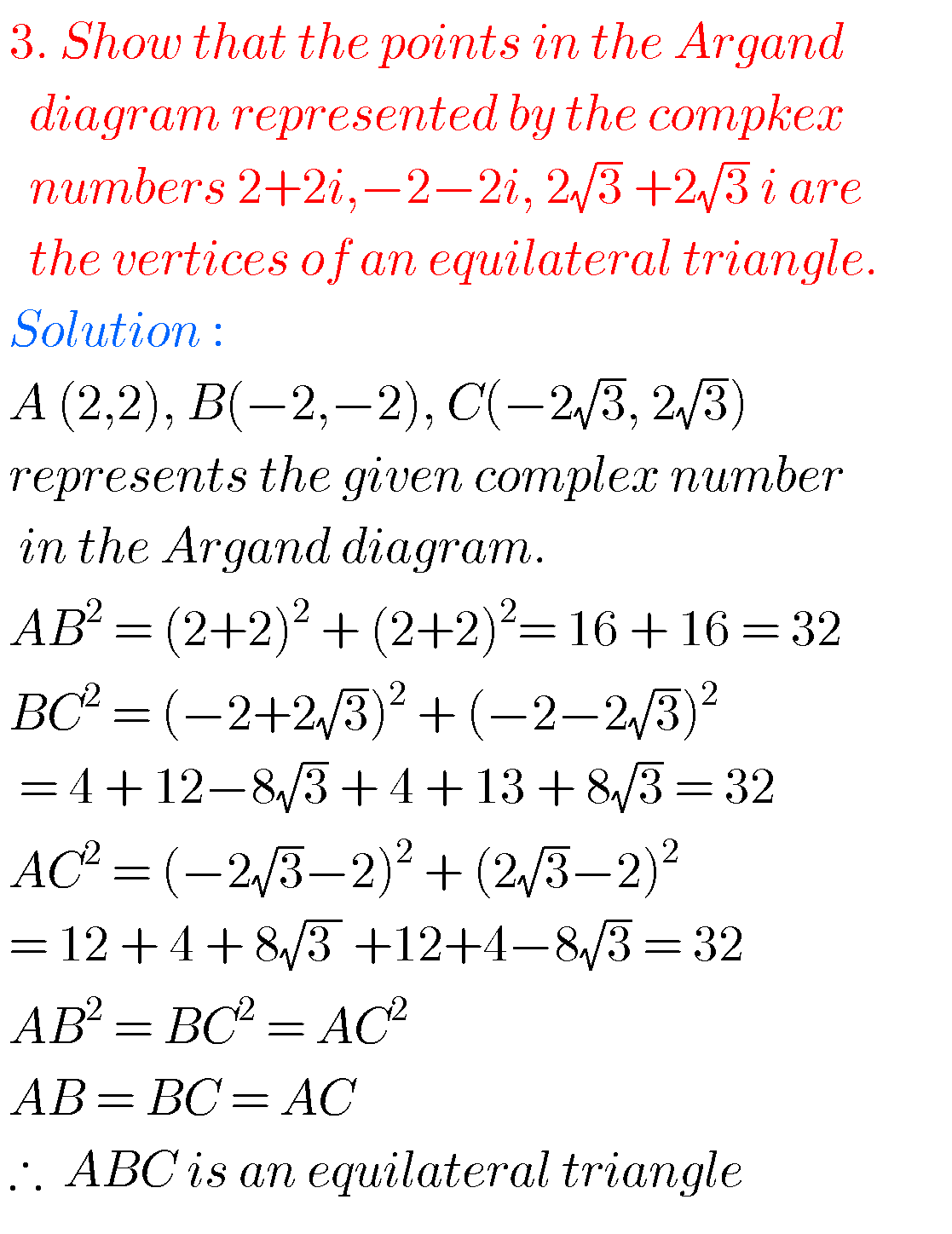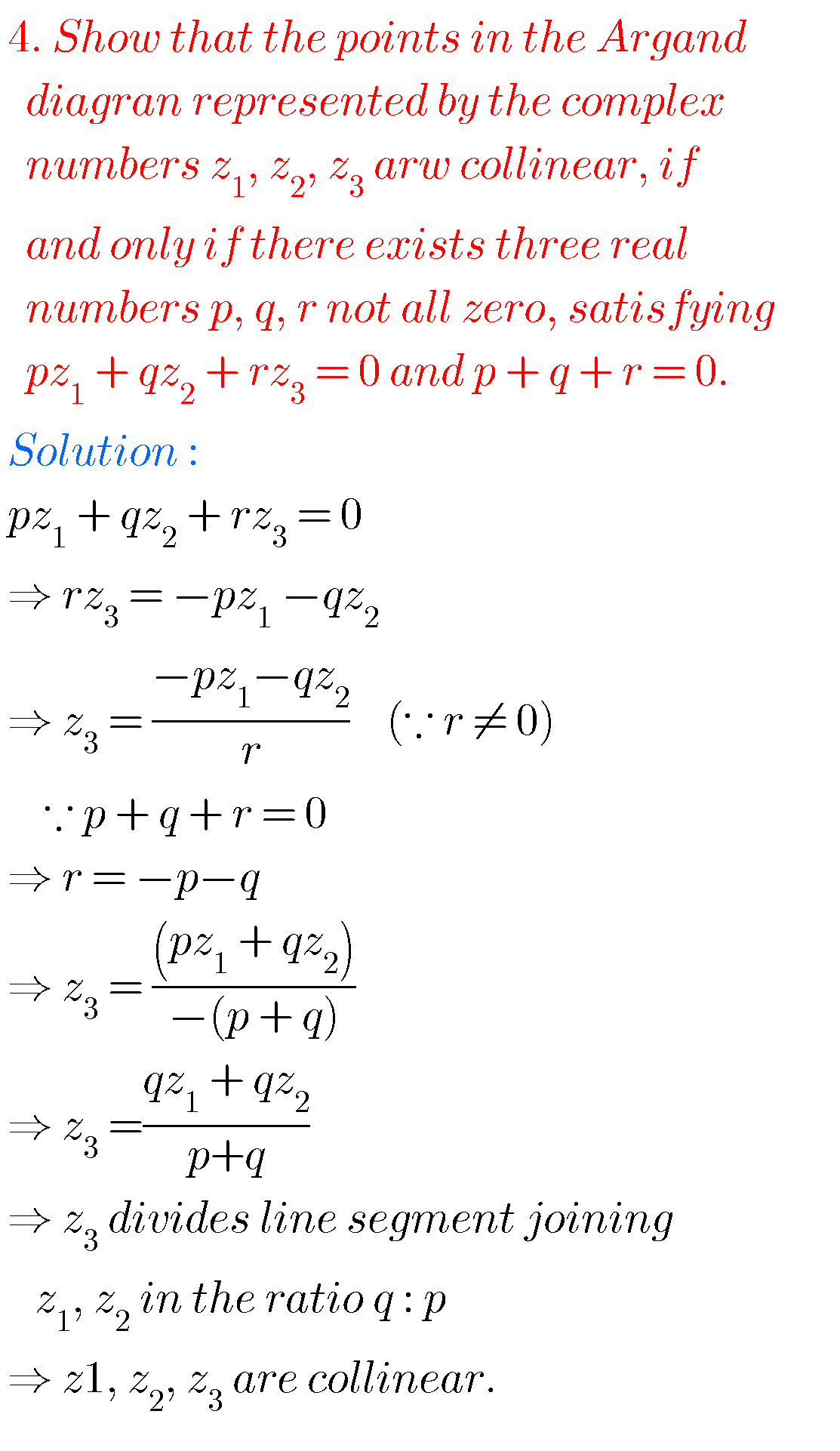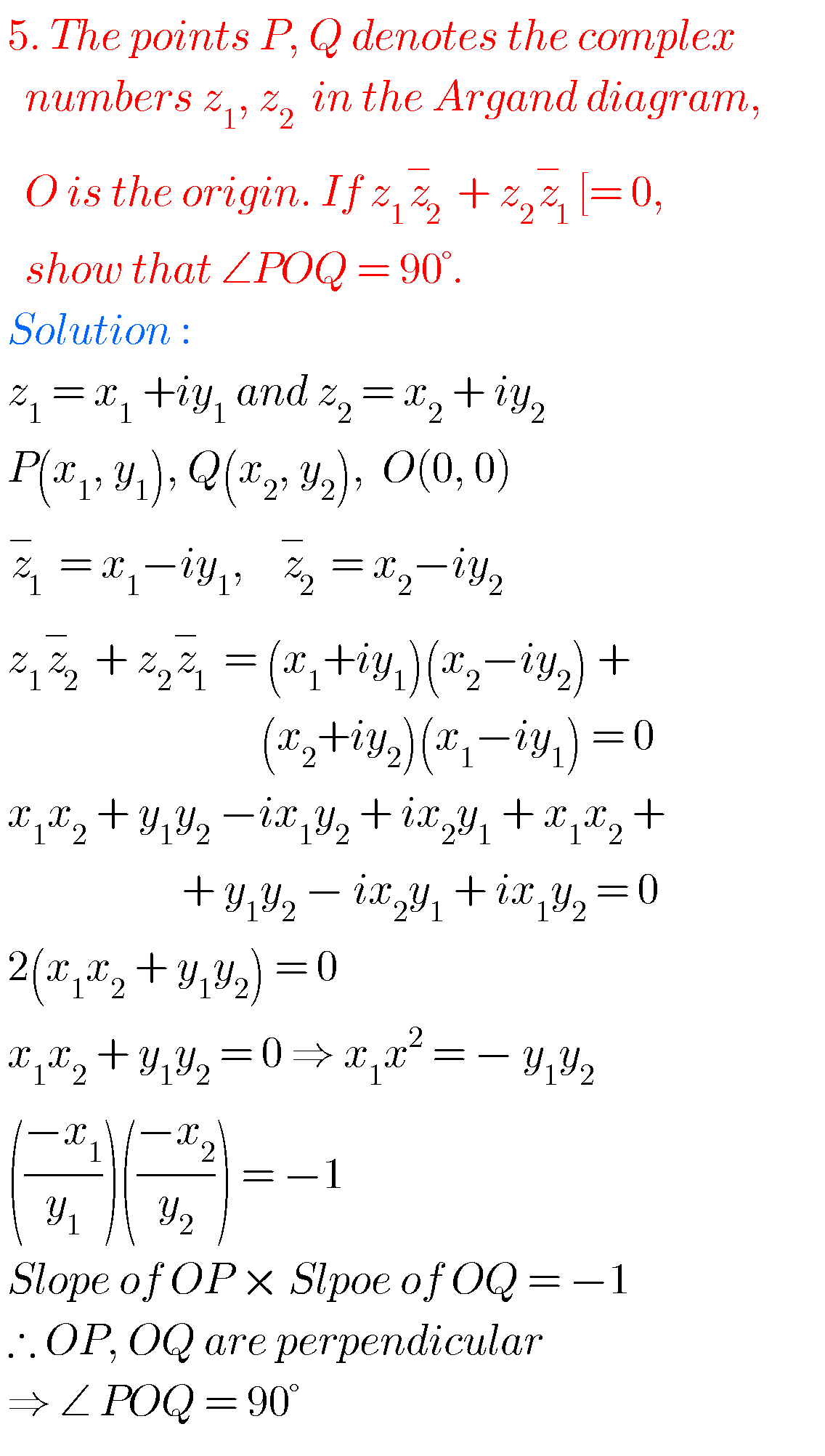Note : Observe the solutions and try them in your own method.You can see the solutions for Inter maths IIA

Complex numbers textbook exercises

4. Theory of Equations textbook solutions

5. Permutations and Combinations text book solutions

6. Binomial Theorem textbook solutions

7. Partial Fractions textbook solutions

8. Measures of Dispersion textbook solutions

9. Probability textbook solutions

10. Random Variables and Probability Distributions textbook solutions

M

### 2 thoughts on “Intermediate maths solutions for Complex numbers”

1.Super

2.Tq and super